# Micromaps host Satellite Design Proposal

 Page 11/11 Date 23.04.2018 Size 1.32 Mb. #46105

## 2.6 Communication

The communications system of the VASCAT transmits telemetry and health information to a ground station and receives commands from a ground station. The VASCAT communications system is based on HokieSat’s communications system with the addition of an AMSAT link.

The uplink portion of the communications system operates at approximately 450 MHz using a loop antenna with a 100KHz bandwidth. The loop antenna is a HokieSat custom design, as shown in Appendix B. The loop antenna is mounted on the nadir panel of the satellite and is connected to an ultra-high frequency (UHF) receiver. The uplink system receives commands from ground stations.5

The UHF receiver used on HokieSat is a modified Tekk model 960LUHF data transceiver as shown in Figure 13. Table 26 gives the receiver specifications.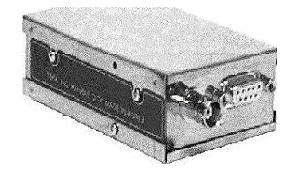Figure 13: Uplink transceiver5

 Type Value FCC ID GOXKS-900/15.22.90 Frequency 430-450MHz Operating Temperature -30 to +60C Voltage 9.6 V Dimensions 3.4”  2.1”  0.9” Weight 5.2 ounces

The communications downlink operates using the S-Band. The downlink system is comprised of a patch antenna and a L3 communications model DST802 transmitter (Figure 14). The frequency of the downlink is between 2,200 and 2,290 MHz while the required bandwidth is approximately 200 kHz. The downlink system transmits telemetry and health information to ground stations at approximately 100 bps. Table 27 shows the specifications on the L3 communications transmitter. 5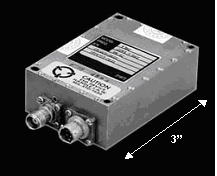Figure 14: Downlink transmitter5

Table 27: Downlink transmitter specifications5

 Type Value Frequency 2.2 –2.3 GHz Power Output 2 W Voltage 28 ±VW Dimensions 2” x 3” x 0.80” Mass 200 g

## 2.6.3 AMSAT

The AMSAT link of the communications system transmits the data produced by the MAPS instrument. The MAPS instrument produces 40 bps of data. Every ten seconds the MAPS instrument requires a time stamp, attitude, position, and velocity of the VASCAT added to the data to be downloaded. Assuming that the satellite data is available as floats, a total of 35.2 bps is added to the link budget. With the addition of the satellite data, the AMSAT link needs to transmit a total of 75.2 bps.19

The UoSAT-OSCAR 22 and the Malaysian-OSCAR 46 spacecraft use the AMSAT as their primary downlink to ground stations. These spacecraft have the capability of a 9,600 bps download rate using a transmitter at 435.12 MHz. The UoSAT-OSCAR 22 spacecraft uses the AMSAT to send grayscale pictures of the earth down to the ground stations. The size of the OSCAR 22 pictures is approximately 109 bytes.2 If VASCAT uses the same method and size for pictures of the Earth's atmosphere, a picture could be downloaded in 10 seconds using the maximum 9,600 bps download rate. The requirements on the AMSAT portion of the communications system needs to be defined further before the specific hardware is selected for use in the VASCAT satellite.

## 2.7 Command and data handling

The command and data handling system of the VASCAT satellite is identical to the HokieSat system. The computer is centered on a Hitachi SuperH RISC Processor, with a 16 MB telemetry buffer, a digital and analog interface subsystem, and a DMA-oriented CMOS camera frame buffer. The orbit average power is 3 W for the computer which. The computer can handle approximately 20 million instructions per second and is radiation hardened to 5 krads. The computer uses VxWorks as a real-time operating system.12

## 2.8 Summary

Chapter two presents the preliminary subsystem designs and configuration for the VASCAT. The systems defined in this chapter include the structure, the ADCS, the power system, the thermal system and the communications system. Each system is developed based on the HokieSat design. The designs presented in Chapter 2 serve as a starting point for further research and development.

## 3.1 Orbits

The main parameters considered in choosing the VASCAT orbit include the amount of Earth coverage the orbit pattern can provide and the lifetime of the satellite in its given orbit. The sensitivity of the MAPS instrument requires a satellite without a propulsion system. The lack of a propulsion system also reduces the complexity of the satellite and reduces operations costs. The success of the mission depends heavily on the satellite’s ability to sustain a three-year lifetime without propulsion. This chapter presents analysis done to determine Earth ground coverage and lifetime predictions.

## 3.1.1 Coverage

The objective of the µMAPS instrument is to perform analysis on samples of the Earth’s atmosphere. Using the maximum amount of points possible in the Earth’s atmosphere is important to the success of the mission. Each one of these points corresponds to a point on the Earth’s surface. Using MATLAB, a coverage analysis is designed to determine the amount of time the µMAPS instrument sees each of these points over a given satellite lifetime. This code is shown in Appendix C.

The MATLAB code uses inputs of the five main orbital parameters: semi-major axis, eccentricity, inclination, argument of perigee, and right ascension of ascending node. It begins by defining a 91 × 361 coverage matrix whose components are all initially zero. The components of this matrix correspond to each point of latitude and longitude on the Earth’s surface. Based on the assumption that the northern and southern hemispheres have symmetrical coverage patterns, only 90 degrees of latitude are used. The program first propagates the input orbit over a given lifetime, in steps of mean motion, to determine the position and velocity of the satellite at any given time during the precession. For each point in time, the code then iterates through a series of targets, each corresponding to one point on the Earth’s surface. These targets cover each point over 90º of latitude and 360º of longitude. The Earth center angle (ECA) is calculated between the satellite and the target and is compared with the angle associated with the field of view of the instrument. The field of view of the instrument is 3º, or approximately 25 km2 on the surface of the Earth.19 If the ECA is greater than the field of view, the satellite is not in view of its target. If the ECA is less than the field of view, then the target is in view and that element of the coverage matrix is incremented by one to show that the target has been viewed for one second. This code iterates over a period of 100 orbits. After 100 orbits, the orbit pattern repeats. Therefore, the coverage over the entire lifetime of the satellite is determined. Parameters such as orbit inclination and altitude alter the range and frequency of coverage. This code is used to compare the coverage of a series of orbits. This orbit property is considered along with other factors to determine the optimal orbit for the VASCAT. This type of data is also used as a concrete model illustrating the capabilities of the mission.

## 3.1.2 Orbit prediction

Lifetime calculations predict the amount of time a LEO satellite remains in orbit before atmospheric drag causes re-entry. The lifetime of a satellite is calculated to decide if the mission goals are fulfilled before end-of-life, and determine optimal orbit parameters. Sources of uncertainty in satellite orbital lifetime prediction are estimated future solar radio flux and geomagnetic activity, modeled atmospheric density, and ballistic factor. The main source of uncertainty in models estimating future atmospheric density at orbital altitude is the solar extreme ultraviolet heat input values. An important problem in mission planning and satellite command & control is accurate prediction of orbital motion.

## 3.1.3 Orbit simulation

An orbit propagator is a mathematical algorithm for predicting the future position and velocity (or orbital elements) of an orbit given some initial conditions and assumptions. There are a wide variety of orbit propagation techniques available with different accuracy and applications. All built-in assumptions must be known before deciding on a propagation scheme.

An accurate atmospheric model computes drag effects using Satellite Tool Kit’s (STK) lifetime prediction feature.1 The gravitational model for the Earth is significantly simplified since the inclusion of the higher order terms does not impact orbit decay estimates.

## 3.1.4 Orbit characteristics

The VASCAT launches into a circular orbit with an initial altitude of 450 km and an inclination of 57 degrees. The desired design lifetime of the satellite is initially three years. A circular orbit obtains homogeneous and complete global coverage of the Earth. An initial altitude of 450 km guarantees a multi-year mission duration even under severe solar activity conditions. The altitude decreases over the mission lifetime due to atmospheric drag. The VASCAT may pass through the solar activity maximum, depending on launch. The predicted natural decay depends on the magnitude of the actual solar activity cycle.

The lifetime tool, available in STK, is used to estimate the amount of time the VASCAT remains in orbit. Lifetime computations are based on complex orbital theory and an accurate environment model. The results are only an estimate. Due to variations in atmospheric density and irregular solar activity, satellite lifetimes are determined with accuracy no better than ±10 %.1 To implement a practical computer lifetime program, some assumptions and simplifications are made that add additional degrees of uncertainty to the final result. Various parameters that effect lifetime include mass, altitude, satellite drag coefficient, satellite drag area, and orbit inclination. Because the VASCAT’s mass is fixed at approximately the 35‑50 kg margin, all calculations are performed with the satellite’s mass fixed at 50 kg.

The most important parameter in determining the lifetime of the VASCAT is initial altitude. Figure 15 shows the complete trend from which an initial orbit altitude is determined to fulfill the mission lifetime.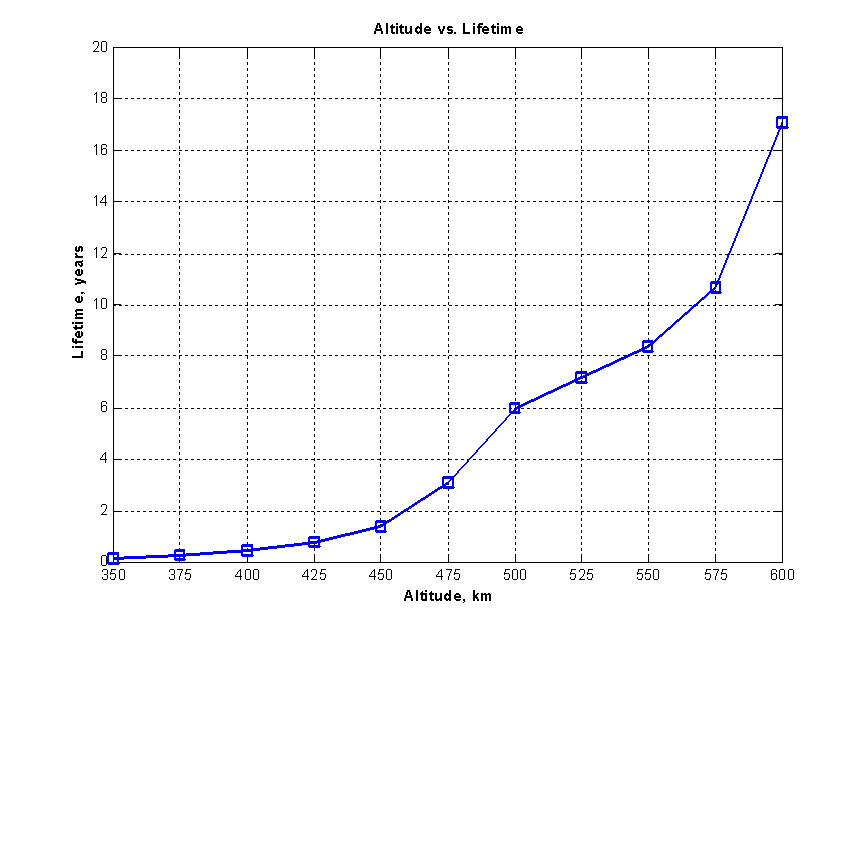Figure 15: Lifetime as a function of altitude

The satellite’s drag coefficient, Cd is between 2.0 and 2.2. However, in calculating the effects of Cd, values are varied from 1 to 3 to more clearly see the trend that Cd has on lifetime. The results are plotted in Figure 16 and Figure 17 for two altitudes.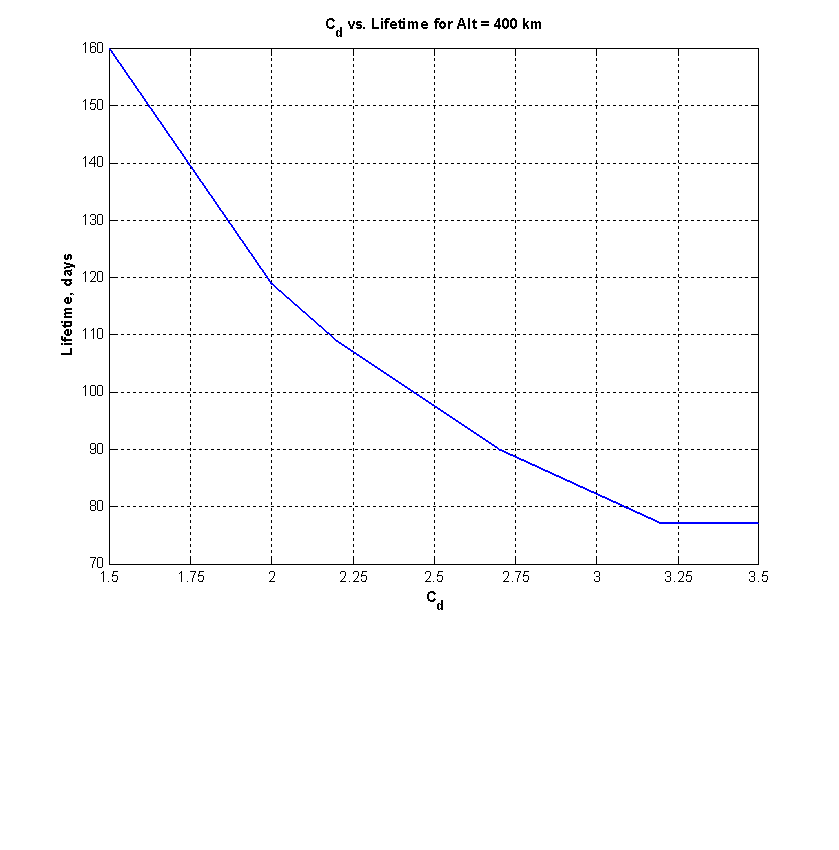Figure 16: Lifetime as a function of drag coefficient for a 400 km altitude orbit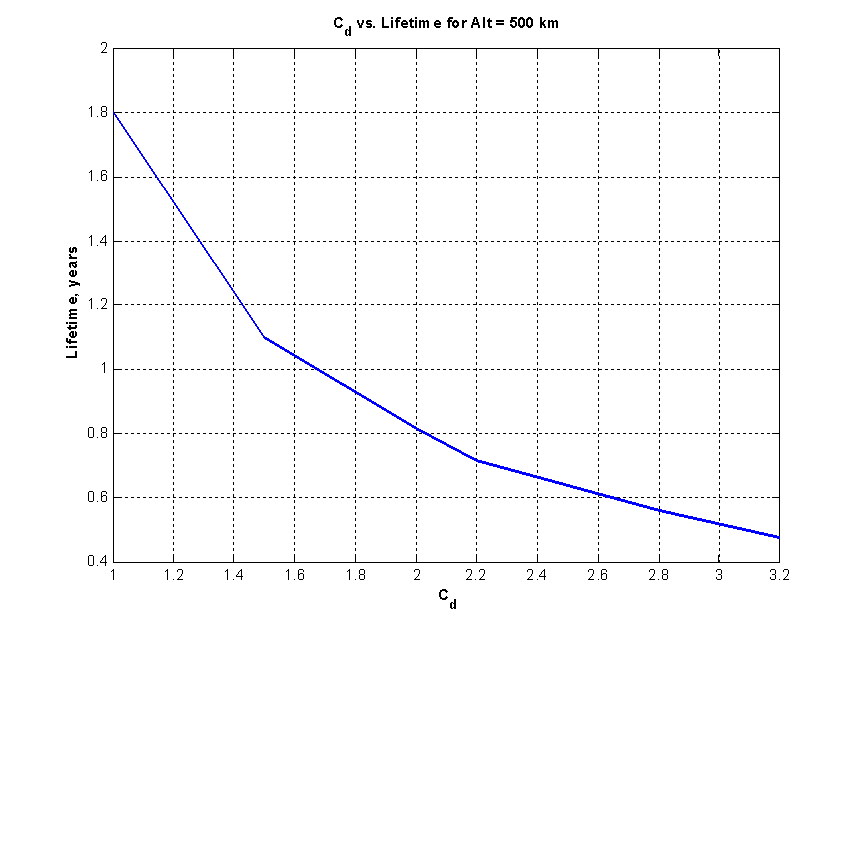Figure 17: Lifetime as a function of drag coefficient for a 500 km altitude orbit

The drag area of the satellite is the mean cross-sectional area of the satellite perpendicular to its direction of travel. The effects of altitude are examined at two altitudes; the results can be seen in Figure 18 and Figure 19.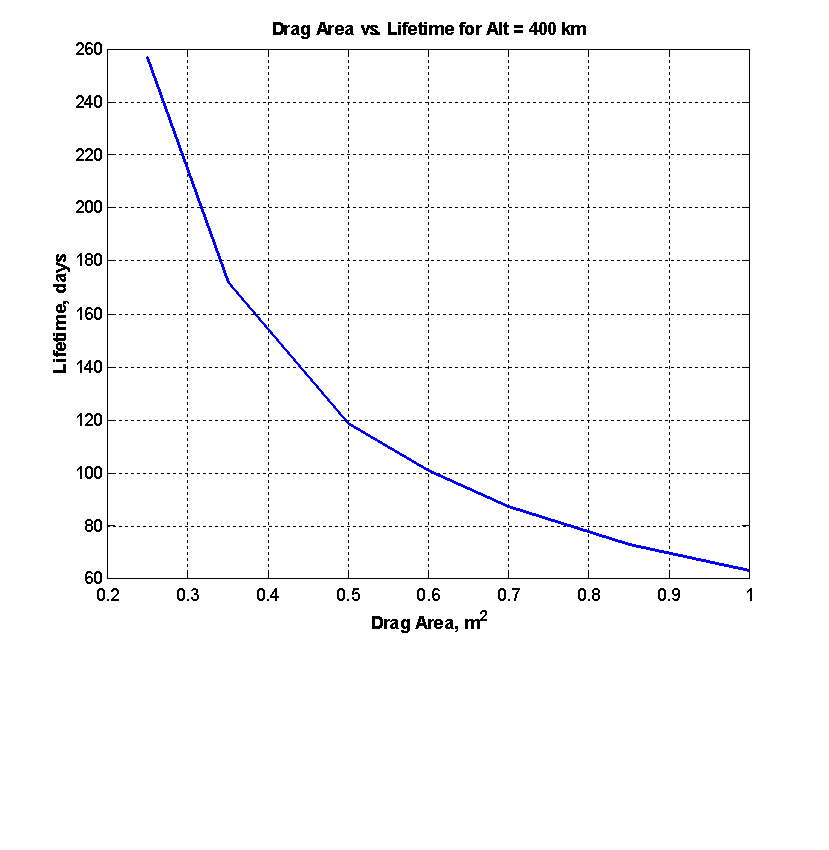Figure 18: Lifetime as a function of drag area for a 400 km altitude orbit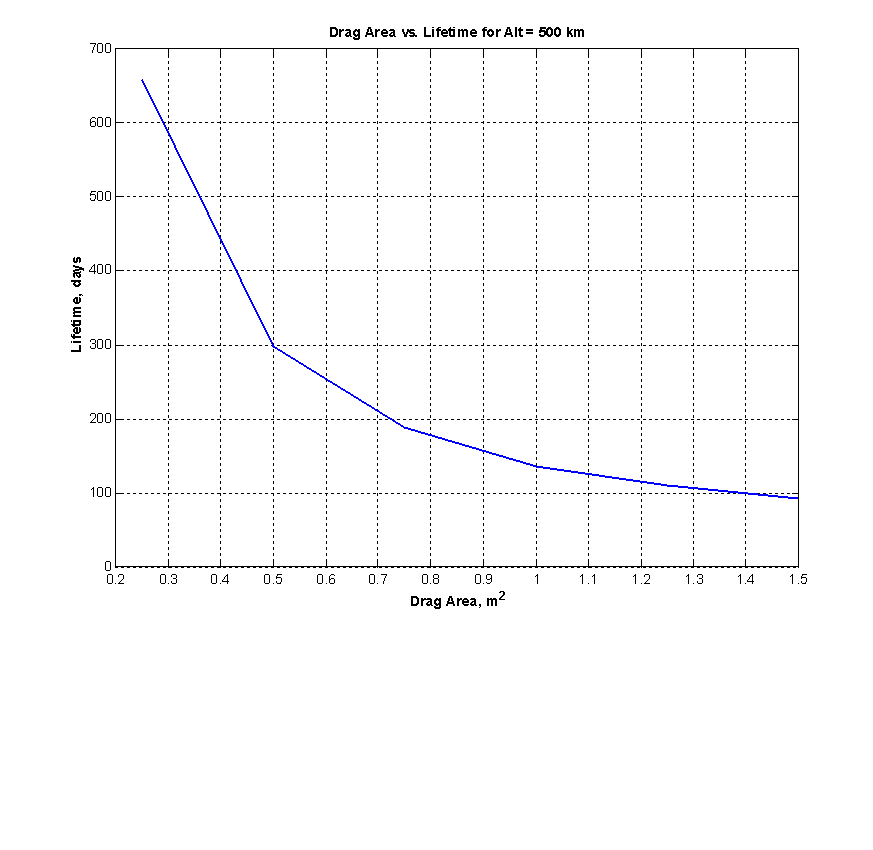Figure 19: Lifetime as a function of drag area for a 500 km altitude orbit

Inclination of the orbit effects the coverage of a satellite. Inclination analysis is performed and displayed in Figure 20.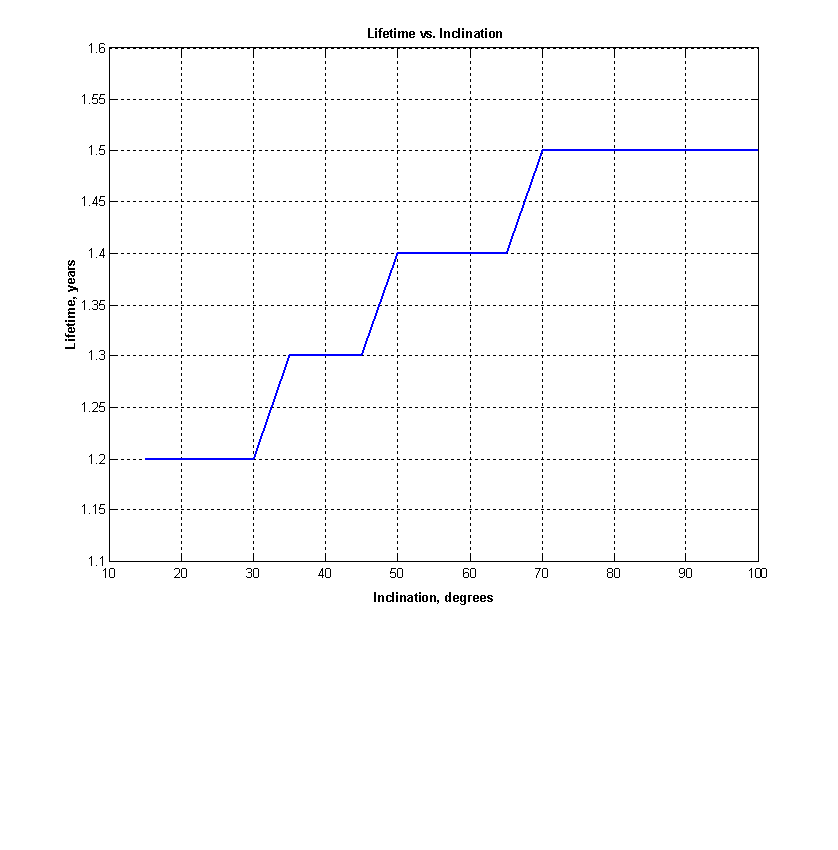Figure 20: Lifetime as a function of orbit inclination

For all calculations the decay altitude is 300 km. The decay altitude is the altitude at which the satellite’s orbit is determined to be decayed. This is the altitude at which lifetime calculations stop.

## 3.2 Summary

Chapter three introduces the two main parameters in choosing an orbit for the VASCAT. A method for predicting Earth ground coverage is presented which is useful in choosing an orbit or predicting mission capabilities. Also, the variations of lifetime with orbit altitude, drag area, drag coefficient, and inclination are presented in chapter three. These variations provide the minimum orbital parameters for the VASCAT. The lifetime information can be combined with the coverage information to determine the optimal orbit for the VASCAT.

## Chapter 4: Cost Analysis

A cost model predicts the overall cost of a project, from initial research and testing to its final end-of-life costs. Cost modeling is used as a method of maximizing performance while remaining within budgetary constraints, rather than estimating the cost of a project with given performance parameters.20

The parametric cost estimation method (PECM) uses mathematical relationships to relate input parameters directly to the cost of the project. The equations used are known as the cost estimation relationships (CER) and are expressed as a function of the cost drivers. Several assumptions are made when using a PECM. The most predominant assumption is that the PECM is based on historical spacecraft mission costs. A specific PECM is limited on the type and size of spacecraft for which it is effective. 20

The PECM used for the VASCAT is similar to an example for scientific small LEO satellites found in the Space Mission Analysis and Design reference. 20 The VASCAT fits into this description for the PECM. Table 28 shows the output of the PECM using parameters from the VASCAT. The total cost of producing the VASCAT is found by summing the values in the right hand column of Table 28. The cost to produce the VASCAT is approximately \$16 million to \$49 million. This cost is greater than HokieSat's budget of \$1.5 million, which does not include testing costs or student labor costs.

NASA's Johnson Space Center provides the public with an online cost estimator for different types of spacecraft. One of the spacecraft options is a scientific satellite with mass as the only input. The mass of the VASCAT is assumed to be 40 kg. The NASA calculator outputs a development and production cost of approximately \$15 million and an operations cost of approximately \$0.5 million per year.13

Table 28: The VASCAT cost estimate using PECM20

 Cost component Parameter x (unit) Input value Subsystem cost (FY00\$K) 1. Payload Satellite total cost (FY00\$K) 33,000 13,100 2. Satellite Satellite bus dry mass (kg) 40 3,510 2.1 Structure Structures mass (kg) 12 722 2.2 Thermal Thermal control mass (kg) 5 351 2.3 Electrical power system Average power (W) 100 315 Power system mass (kg) 7 1,590 Solar array area (m2) 1.3 3,260 Battery capacity (A-hr) 5 2,030 BOL power (W) 130 3,750 EOL power (W) 110 3,480 2.4a Telemetry tracking & command TT&C/DH mass (kg) 3 536 Downlink data rate (Kbps) 100 2,570 2.4b Command & data handling TT&C + DH mass (kg) 3 726 Data storage capacity (MB) 16 2,800 2.5 ADCS ADCS dry mass (kg) 14 3,040 Pointing accuracy (deg) 5 1,520 Pointing knowledge (deg) 1 2,640 2.6 Propulsion Satellite bus dry mass (kg) N/A 0 Satellite volume (m3) N/A 0 Number of thrusters N/A 0 3. Integration, assembly, & test Satellite total cost (FY00\$K) 33,000 4,570 4. Program Level Satellite total cost (FY00\$K) 33,000 7,530 5. Ground support equipment Satellite total cost (FY00\$K) 33,000 2,170 6. Launch & orbital operations support Satellite total cost (FY00\$K) 33,000 2,010

The total cost of the VASCAT ranges from \$16 million to \$49 million. Each of these estimates is examined, to find the areas that disagree with one another, to determine which of these cost estimates is more accurate. This comparison is difficult to complete because the details of the NASA cost estimator are proprietary and not available for public use.

## Chapter 5: Summary, Conclusions, and Remaining Work

The satellite design VASCAT detailed in this paper is a preliminary design incorporating the MAPS instrument into a host spacecraft. The VASCAT design, based on Virginia Tech’s existing nanosatellite HokieSat, supports the MAPS instrument with a minimum mission lifetime of one year.

The preliminary design of the required subsystems is described in this report. Component level definitions are given for the ADCS, thermal, and power subsystems. The VASCAT is configured for body-mounted solar cells and room to house the instrument and all other subsystem components. The structure design incorporates the MAPS instrument’s existing mounting feet. Preliminary structural analysis is used to size the primary structure. The orbital analysis presented lays the groundwork for determining a more definite orbit to adequately complete the mission. The satellite is designed to meet the desired lifetime requirements of one year without propulsion. The addition of a digital camera is not discussed in detail, but it has been considered by all subsystems and can fit into the VASCAT design.

The AMSAT frequency is proposed to allow high school students to participate in the VASCAT mission. This frequency downloads data from both the MAPS instrument and the proposed digital camera. The camera takes pictures during data collection. The AMSAT downlink needs to be further defined to accomplish the download of atmospheric pictures and the secondary educational requirements.

All subsystem designs are incomplete. Further knowledge of the AMSAT hardware is required to fully define its use. The interior of the VASCAT, including component placement and wiring paths, must be configured. A launch vehicle must be chosen for further structural analysis and design. Most importantly, a design and fabrication budget is required to continue high level subsystem design.

## References

1. Analytical Graphics, Inc. “Analytical Graphics, Inc.” 2002. (24 March, 2002)

2. AMSAT. "AMSAT From A to Z." 2002. (4 May, 2002)

3. C&R Technologies, Inc. "SINDA/FLUINT 4.4 manual." 2002. (2 May, 2002)

4. Cengel, Y.A. Introduction to Thermodynamics and Heat Transfer. Irwin/Mcgraw-Hill. Botson, Massachusetts. 1997.

5. Findlay, S. ION-F Flight Communications Design Specification. University of Washington, Seattle, Washington. Issue date: 14 September, 2000.

6. Gilmore, D.G. and Bello, M. (editors). Satellite Thermal Control Handbook. The Aerospace Corporation Press. El Segundo California. 1994.

7. Harvey, A.C. HokieSat Interface Control Document, Revision VT ICD C-2. Virginia Polytechnic Institute and State University, Blacksburg, Virginia. Issue date: 2 November, 2000.

8. HokieSat webpage, webmaster Jana L. Schwartz. Updated 14 March, 2002.

9. Incropera, F.P. and DeWitt, D.P. Fundamentals of heat and mass transfer, fourth edition. School of Mechanical Engineering, Purdue Univ. John Wiley and Sons. New York. 1996.

10. Isakowitz, S.J., Hopkins, J.P. and Hopkins, J.B. International Reference Guide to Space Launch Systems: Third Edition. AIAA Publishing. Reston, Virginia. 1999.

11. Ithaco. “Ithaco Home Page.” 1997. <http://www.ithaco.com/> (28 April, 2002)

12. Jensen, J.D. and Swenson C.M. Command and Data Handling Subsystem Design for the Ionospheric Observation Nanosatellite Formation. Utah State University, Logan, Utah. Issue date: 28 June, 2000.

13. Johnston Space Center. “JSC Homepage.” 2002. <http://www.jsc.nasa.gov/> (20 April, 2002)

14. Sanyo North America Corporation. “Nickel-Metal Hydride Battery HR-4/3 FAU 4500.” 2001. (26 April, 2002)

15. Sarafin, T. Spacecraft Structures and Mechanisms from Concept to Launch.
Torrance: Microcosm Press. 1995.

16. Spectrolab. "Spectrolab Products." 2000. (21 March, 2002)

17. Systron Donner. “Systron Donner Inertial Division.” 1998. <http://www.systron.com/> (20 April, 2002)

18. Valley Forge Composite Technologies. “VFCF: Valley Forge Composite Technologies.” 2001. <http://www.vfct.com/> (20 April, 2002)

19. Walberg, G., Reichle, H.G. and Morrow, W.H. Design and Testing of MicroMAPS Gas Filter Correlation Radiometer. 21 January 1999.

20. Wertz, J.R. and Larson, W.J. (editors). Space Mission Analysis and Design 3rd Edition. Microcosm Inc. El Segundo, California. 1999.

## Appendix A: MATLAB Power Code

% This function takes the height and major diameter of a hexagonal prism

% and returns the projected area of the eight faces.

% This function returns a matrix eight by N (time step) with projected areas

% for the faces in the rows

% and the columns are different instances in the orbit.

function pete = bidwell(height,diameter)

W = 0*pi/180;

inc = 50*pi/180;

si = [1 0 0]';

a = 6778;

mu = 3.986*10^14;

Gs = 1358;

sce = 0.20;

Ld = 0.02;

life = 1;

Id = 0.88;

n = sqrt(3.986*10^5 / a^3);

Period = 2*pi/n;

Npoints = 900;

tspan = linspace(0,Period,Npoints);

facesb = faces(height,diameter);
R13 = R1(inc)*R3(W);

Roop = [0 1 0; 0 0 -1; -1 0 0];

for ti = 1:1:Npoints

% Calculation of True Anomaly

u = n * (tspan(ti) - 0);

% Rotations

Roi = Roop*R3(u)*R13;

Rbo = eye(3,3); %Orbital frame aligned with body frame

Rbi = Rbo * Roi;

sb = Rbi * si;

rper = [a*cos(u) a*sin(u) 0]';

ri = Roi*rper;

alpha = atan(6378/norm(ri));

%Eclipse angle

if u <= alpha

%If u is less than or equal to alpha, then the satellite is eclipsed

eclipse=0;

%eclipse=0 when satellite is eclipsed and eclipse=1 all other times.

elseif u >= 2*pi-alpha

eclipse=0;

else

eclipse=1;

end

for i = 1:8

costheta(i) = sb' * facesb(1:3,i);

if costheta(i) <= 0

costheta(i) = 0;

end

Aproj(i,ti) = costheta(i) * facesb(4,i) * eclipse;

%Projected Area

end

end
Po = Gs*sce;

Id = 0.77*Id; %Inherent Degradation

Ld = (1-Ld)^life; %Life Degradation

Pbol = Po*Id; %Power/area Beginning of life

Peol = Ld*Pbol; %Power/area End of life

power = Peol * Aproj;
sumpower = sum(power,1);
te = 1.7341*10^3;

td = 3.9130*10^3;

tt = linspace(0,td,Npoints);
for k=2:Npoints

E(k) = (tt(k)-tt(k-1))*sumpower(k);

end
Eproduced = sum(E,2)

Eneeded = 275789.1388 %Determined from sum of eclipse energy

%Ouput maximum area seen by sun in predicted orbit.

fprintf('Thermal Areas: \n')

for j = 1:8

one(j) = max(Aproj(j,:));

fprintf(2,'Max Area of face',j);

fprintf(1,' : %f\n',one(j));

end
%Plots
figure(1)

plot(tspan,Aproj)

title('Projected Area from all the faces vs. time')

xlabel('time (s)')

ylabel('Power (W)')

legend('face 1','face 2','face 3','face 4','face 5','face 6','Zenith','Nadir')

figure(2)

plot(tspan,power)

title('Power from all the faces vs. time')

xlabel('time (s)')

ylabel('Power (W)')

legend('face 1','face 2','face 3','face 4','face 5','face 6','Zenith','Nadir')

figure(3)

plot(tspan,sumpower)

title('Sum of Power from all the faces vs. time')

xlabel('time (s)')

ylabel('Power (W)')

## Appendix B: HokieSat Loop Antenna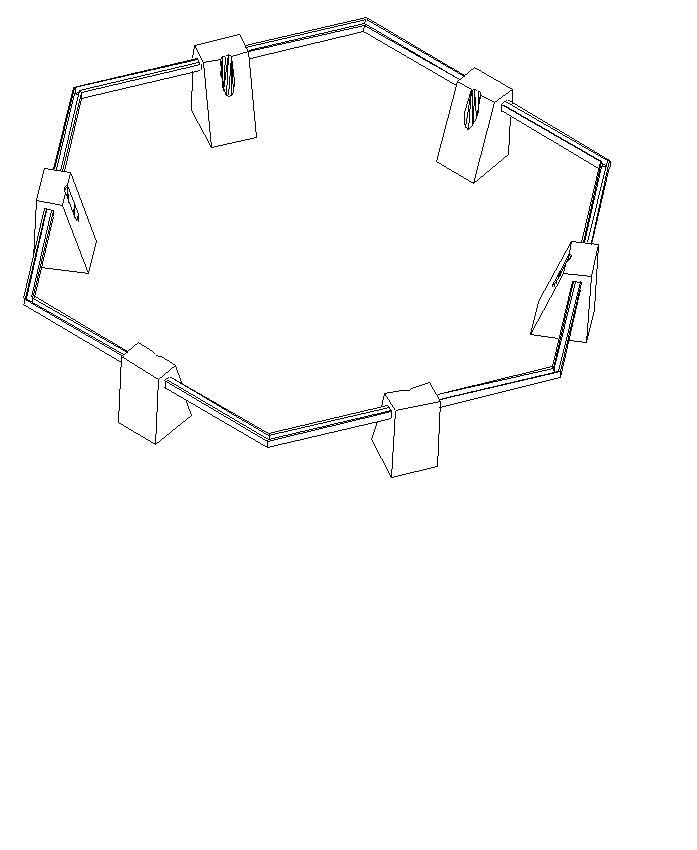Figure 21: Loop antenna assembly from HokieSat drawing package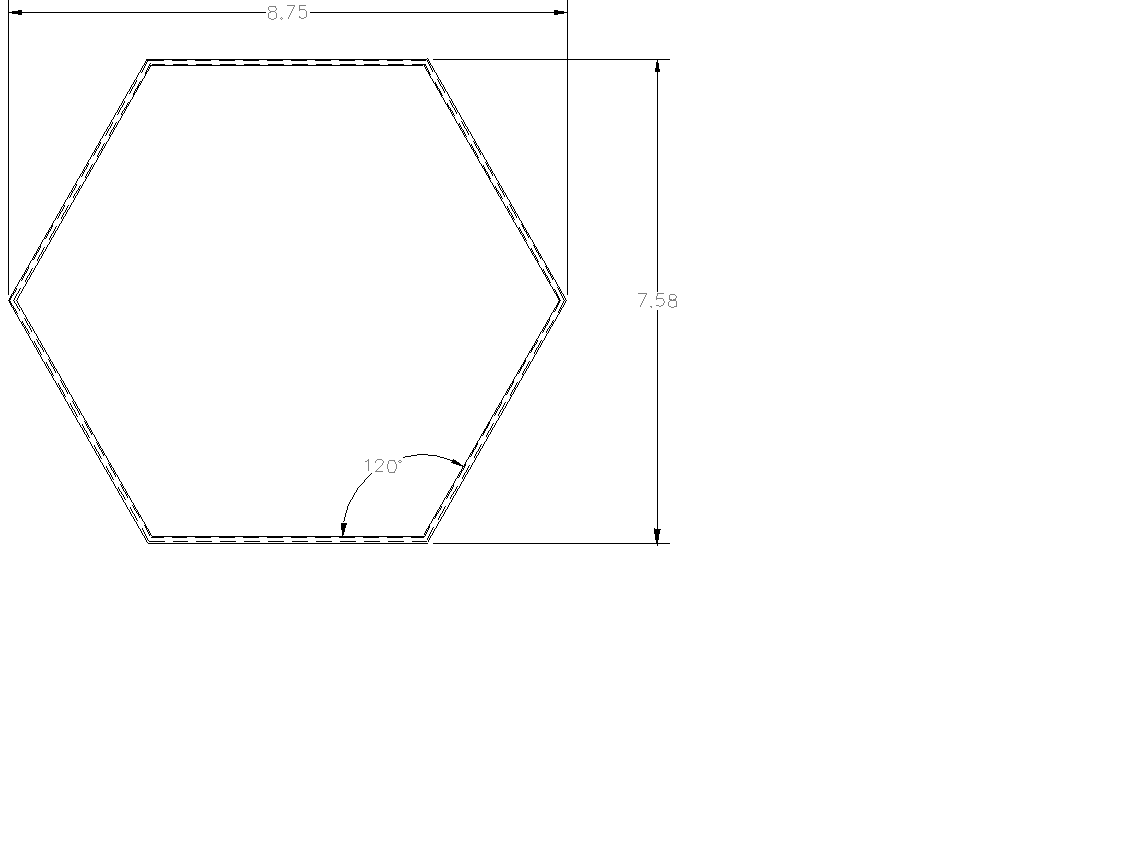Figure 22: Copper tube loop from HokieSat drawing package

## Appendix C: MATLAB Earth Ground Coverage Code

% This code takes the orbital elements and propagates the orbit to determine the amount % of time a given point on Earth’s surface is in view
% Input parameters
mu=3.986004418*10^5; % Earth's gravitational constant (km^3/s^2)

cf=pi/180; % conversion from degrees to radians

Re=6378.13649; % radius of the Earth in nmi

c=2.99792458*10^5; % speed of light (km/s)

H=500; % satellite altitude (km)

a=Re+H; % semi-major axis (km)

e=0; % eccentricity

i=57*cf; % inclination

w=0*cf; % argument of perigee

an=0*cf; % longitude of ascending node

p=a*(1-e^2);

h=sqrt(mu*p); % momentum (km^2/s)

period=2*pi*sqrt(a^3/mu); % orbital period (s)

n=sqrt(mu/(a^3)); % mean motion (rad/s)

% calculate FOV

rho=asin(Re/(Re+H))/cf; % angular radius of the earth

nadir=1.5; % max off-nadir angle of instrument field of view

elevation=acos(sin(nadir*cf)/sin(rho*cf))/cf; % cooresponding elevation angle

FOV=90-elevation-nadir; % field of view of instrument on Earth's surface
lat=0;

q=1;

COV=zeros(91,361);
% **TARGETS**
while lat<=90

%latitude of the target

lon=0;

s=1;

while lon<=360

%longitude of the target

if lat<0

phi=(90-lat)*cf;

z=Re*cos(phi);

elseif lat>=0

phi=(90-lat)*cf;

z=Re*cos(phi);

end

theta=lon*cf;

x=Re*sin(phi)*cos(theta);

y=Re*sin(phi)*sin(theta);

target=[x y z]; % target radius vector in ECF

tmag=norm(target); % magnitude of target vector in ECF

f=0; % true anomaly

M=0; % mean anomaly

j=1;

while M<=100*2*pi

t=M/n;

o(j)=an+7.292123517*(10^-5)*t; % right ascension of ascending node

% angular velocity of Earth = 2* pi rad / 23.934444 hr = 7.292123517E-5 rad/s

k=2;

E(1)=100; % E=eccentric anomaly

E(2)=200;

if e==0

E=M;

elseif M==0

E=0;

else

while ( ( (E(k-1)/E(k))<=0.999) | ( (E(k-1)/E(k) )>=1.001) )

E(k+1)=E(k)-((E(k)-M-e*sin(E(k)))/(1-e*cos(E(k))));

k=k+1;

end

E=E(end);

end

if E<=pi

f=acos((e-cos(E))/(e*cos(E)-1));

else

f=-acos((e-cos(E))/(e*cos(E)-1));

end

% calculate radius and velocity in ECF

rsca=p/(1+e*cos(f)); % scalar radius (perifocal)

vr=sqrt(mu/p)*e*sin(f); % perpendicular velocity (perifocal)

vt=h/(rsca); % tangential velocity (perifocal)

% transformation matrix from perifocal to ECF

Ar11=cos(o(j))*cos(w+f)-sin(o(j))*cos(i)*sin(w+f);

Ar21=sin(o(j))*cos((w+f))+cos(o(j))*cos(i)*sin(w+f);

Ar31=sin(i)*sin(w+f);

Ar=[Ar11 Ar21 Ar31];

% transformation matrix from perifocal to ECF

At11=-cos(o(j))*sin(w+f)-sin(o(j))*cos(i)*cos(w+f);

At21=-sin(o(j))*sin(w+f)+cos(o(j))*cos(i)*cos(w+f);

At31=sin(i)*cos(w+f);

At=[At11 At21 At31];

rsat=rsca*Ar; % satellite radius vector in ECF

smag=norm(rsat); % magnitude of satellite radius

vector in ECF (km)

vsat=vr*Ar+vt*At; % velocity vector in ECF

vmag=norm(vsat); % magnitude of velocity vector (km/s)

% find whether the target is in view of the satellite

ECA=(180/pi)*acos((dot(rsat, target)) / (smag*tmag)); % earth center angle

(degrees)

% **RESULTS**

if ECA>=FOV

anamoly(j)=f;

else

R=target-rsat; % resultant vector (satellite to

target)

Rmag=norm(R); % magnitude of the resultant vector

anamoly(j)=f; % vector to store f values

Mdeg=M*180/pi;

COV(q,s)=COV(q,s)+1;

end

j=j+1;

M=M+n;

end

s=s+1;

lon=lon+1;

end

q=q+1;

lat=lat+1;

end

COV

longitude=linspace(0,360,361)

latitude=linspace(0,90,91)

surf(longitude, latitude, COV)

xlabel('longitude')

ylabel('latitude')

zlabel('Seconds of coverage')

## Appendix D: MATLAB Disturbance Torque and Attitude Actuator Sizing Code

% Constants

mu = 3.986012e5; % gravitational constant, km^3/s^2

Fs = 1367; % solar constant, W/m^2

c = 3e8; % speed of light, m/s

q = 0.6; % reflectance factor

M = 7.96e15; % magnetic moment, tesla-m^3

% Spacecraft Properties

Iy = 4.356; % kg-m^2

Iz = 1.875; % kg-m^2

As = 0.6*0.3; % surface area, m^2

cps = 0.165; % location of the center of solar pressure, m

cg = 0.1; % location of the center of gravity, m

cpa = 0.165; % location of the center of pressure, m

D = 1; % magnetic dipole, A-m^2

Cd = 2.5; % drag coefficient
% Orbit Properties

R = 6778; % km

V = sqrt(mu/R); % km/s

OrbitalPeriod = 2*pi*sqrt(R^3/mu); % s

Rho = 2.72e-12; % kg/m^3
% Mission Constraints

AttAccuracy = 2.5; % attitude accuracy, degrees

MaxThetaNadir = 45; % maximum angle from nadir, degrees
% ------------------------------------------------------------

% Worst case disturbance torques

%

Tg = 3*mu/(2*R^3)*abs(Iz-Iy)

F = Fs/c*As*(1+q);

Tsp = F*(cps-cg)
% Magnetic Field

B = 2*M/(R*10^3)^3;

Tm = D*B
% Aerodynamic

Fa = 0.5*Rho*Cd*As*V^2;

Ta = Fa*(cpa-cg)
% Total

Td = Tg+Tsp+Tm+Ta

%

% ------------------------------------------------------------

% ------------------------------------------------------------

% Momentum storage in reaction wheel

%
hReaction = Td*OrbitalPeriod/4*0.707
%

% ------------------------------------------------------------

% ------------------------------------------------------------

% Momentum storage in momentum wheel

%
hMomentum = Td*OrbitalPeriod/4/(AttAccuracy*pi/180)
%

% ------------------------------------------------------------